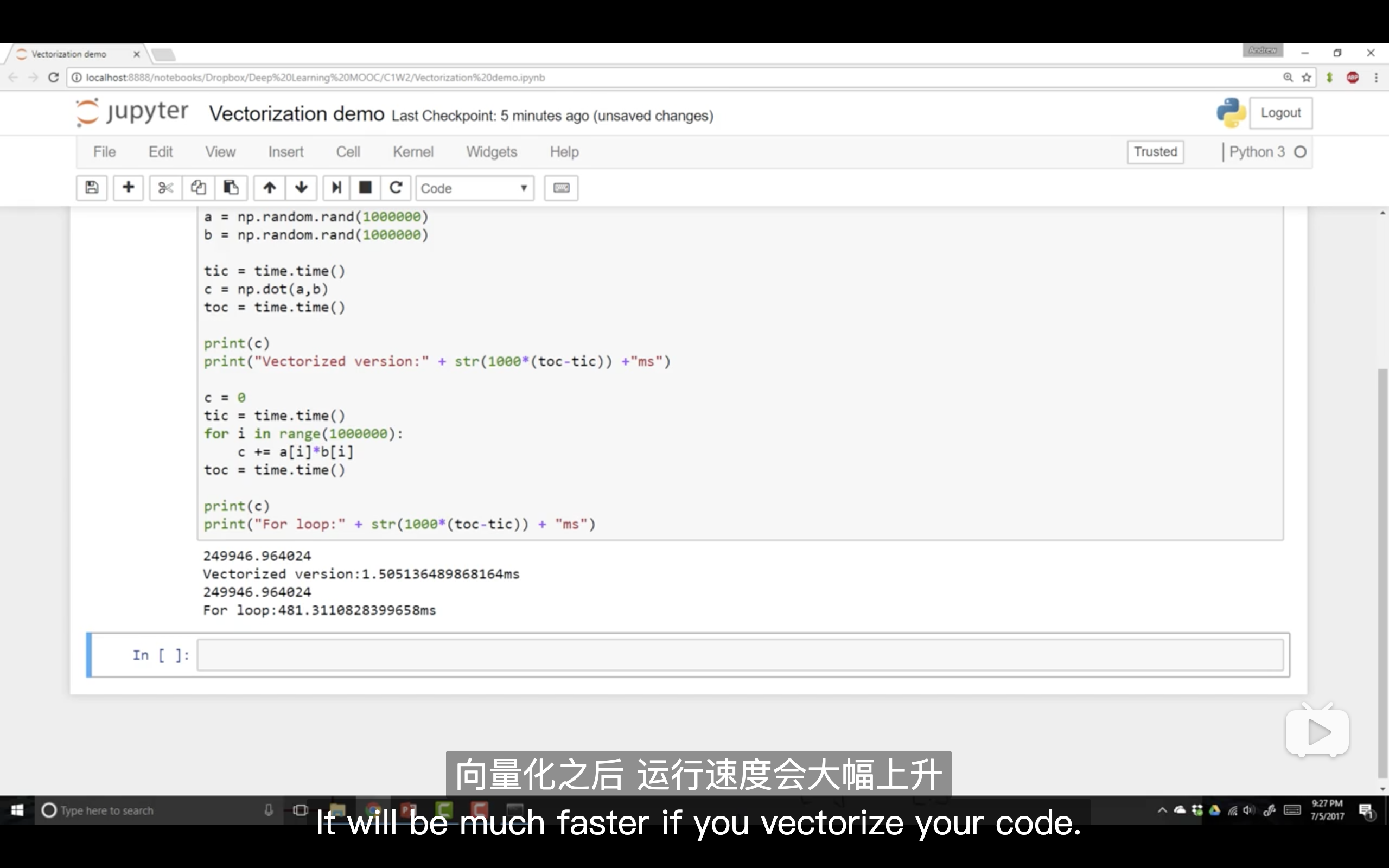# 吴恩达深度学习课程学习笔记第三周

2021/8/20 20:53:25 浏览：

2.7计算图：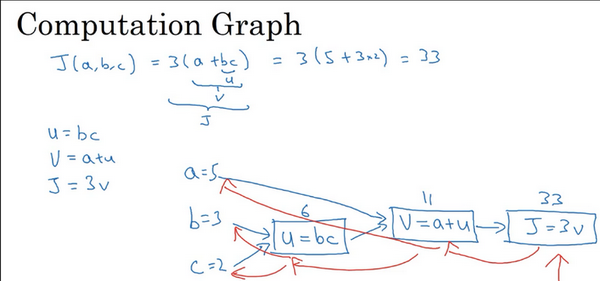Logistic 回归的梯度下降法：

w和b的修正：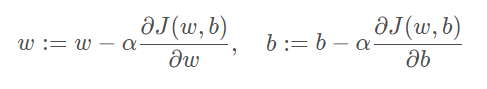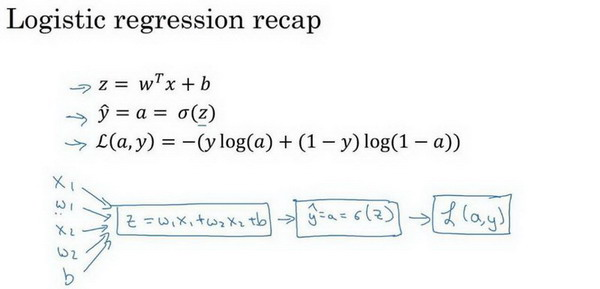求得：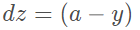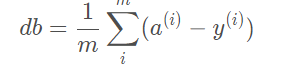m 个样本的梯度下降：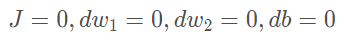J=0;dw1=0;dw2=0;db=0;
for i = 1 to m
z(i) = wx(i)+b;
a(i) = sigmoid(z(i));
J += -[y(i)log(a(i))+(1-y(i)）log(1-a(i));
dz(i) = a(i)-y(i);
dw1 += x1(i)dz(i);
dw2 += x2(i)dz(i);
db += dz(i);
J/= m;
dw1/= m;
dw2/= m;
db/= m;
w=w-alpha*dw
b=b-alpha*db

向量化：

所以如果使用非向量化方法去计算，代码如下：

x
z=0
for i in range(n_x)
z+=w[i]*x[i]
z+=b

z=np.dot(w,x)+b这是向量化计算的方法，非常快。